# GRE Division, Mixed Numerals, and NegativesThis is a post to clarify a potentially confusing passage in the OG.  In the GRE OG 2e, on p. 134, as well as in ETS’s GRE Mathematical Conventions PDF on p. 5, point 9, we find a paragraph discussing quotients and remainders that utilizes the formula n = qd + r. This formula pertains to the situation where an integer n is divided by an integer d. q represents the quotient, and r represents the non-negative remainder. The paragraph then goes on to discuss the results of the following expressions: 20 ÷ 7, 21 ÷ 7, and −17 ÷ 7.

Well, using their formula, we can verify those examples:

a) 20 = (2)(7) + 6

b) 21 = (3)(7) + 0

c) –17 = (–3)(7) + 4

All true.  So far so good.  The problem comes when we want to use all of this to convert an improper fraction to a mixed numeral.  The guiding formula is what I will call the “Division Equation”: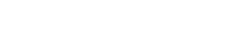This equation is true 100% of the time.  With example (a), if we just plug everything into this formula we magically change the improper fraction into a mixed numeral.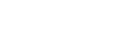Voila! The correct mixed numeral magically results.  With example (b), we don’t need to worry about mixed numerals, because the divisor goes into the dividend evenly, and thus the result of the division is an integer: 21/7 = 3.  With example (c), things get tricky: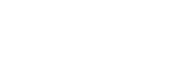The Division Equation above still works, but for negatives, we can’t just dump the numbers in and magically get the correct negative mixed numeral result.  This has to do with the way ETS has chosen to define remainders for negative numbers.  Let’s explore this.

## Remainders for negative numbers

In the passage from p. 134 quoted above, every math book on planet Earth, and probably most written by aliens on other planets, would agree 100% with what ETS said about quotients and remainders for positive numbers.  All that is absolutely undisputable.  The vast majority of math books deliberately ignore the entire topic of what happens to quotients and remainders with negative numbers, because of the mathematical trade-offs that arise.  Most math books have the good sense to avoid this troublesome topic, but ETS brashly charges in and firmly establishes a position.  (To follow knowledge like a sinking star beyond the utmost bound? or, fools rush in where angels fear to tread?)

The disadvantage of defining remainders of negative numbers in this way is that makes finding negative mixed numerals more difficult: we can no longer use the Division Equation — we’ll get back to that point below.  The advantage is: when you divide by a certain divisor, say 7, and talk about all the numbers that have a remainder of 4, those numbers are equally spaced on the number line in both the positive and negative direction.  The numbers which, when divided by 7, have a remainder of 4 are:Notice that –17 appears in that pattern, and follows the same pattern as the familiar positive numbers which, when divided by 7, have a remainder of 4.  It’s very important: if ETS mentions a dividend which, when divided by 7, has a remainder of 4, don’t automatically assume the dividend is positive: it could be negative, and it would follow this sort of pattern.

## Thoughts on mixed numerals

What exactly is a mixed numeral?  That is to say, what do we mean when we write, say: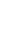What this really means is: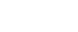In other words, any mixed numeral implicitly contains addition between the integer and the fraction.  That is the BIG idea of mixed numerals.

It’s relatively easy to see that, for positive numbers, the Fraction Equation will result in a whole number quotient plus a fraction less than one, which automatically fits the pattern for a mixed number.

What’s going on with a negative mixed number? Well, that’s a little different: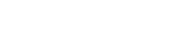The negative sign in front of the whole mixed number applies to both terms and distributes, so you get subtraction instead of addition.  If we are using ETS’ definition of remainder for negative numbers, then the Fraction Equation still works, but it doesn’t help us convert a negative improper fraction to a negative mixed number, because it results in adding the fraction instead of subtracting it.  Again, this part is the disadvantage of the convention ETS is following on this particular topic.  The Fraction Equation, so helpful for converting positive improper fractions to positive mixed numerals, is ostensibly useless in helping us analogously with negative numbers.

## Negative mixed numerals

The question arises, then: how do we convert a negative improper fraction to a negative mixed numeral?  For example, suppose we have the fraction –17/7.  Again, as the OG p. 134 tells us, “when –17 is divided by 7, the quotient is –3, and the remainder is 4.”  That will be consistent with the Fraction Equation, but, as we have seen above, that in turn will not lead to a correct mixed numeral.  Therefore, everything OG says about quotients and remainders for negative number is useless if what we want to find is a negative mixed numeral.  What do we do?

Actually, what you do is remarkably simple: pretend the negative sign isn’t there for the moment, and just convert the positive improper fraction to positive mixed numeral!

Now, we are dividing +17 by 7: of course, the quotient is 2 and the remainder is 3, so the Fraction Equation conveniently gives us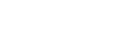So far, so good.  As a result of working with the positive numbers, we know: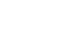But what we wanted was –17 divided by 7, not +17 divided by 7.  How do we get that?  We get that simply by multiplying the previous equation by a negative sign on both sides.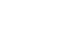Again, to convert negative improper fraction to a negative mixed numeral: just pretend the improper fraction is positive, do the conversion with positive numbers, and then simply make the output negative when you are done.

## Practice questions## Practice question solutions

1) Here, we must follow the ETS convention.  According to this convention, when a negative number is divided the remainder must be positive.  What is the multiple of 6 that is less than –23 and closest to –23?  That would be  –24, so 6 goes into  –23  negative four times, with a remainder of (–23) – (–24) = +1.  This checks out with the ETS’s equation: n = –23, d = 6, q = –4, and r = 1, and it’s true that

–23 = (6)( –4) + 1

So the quotient is –4 and the remainder is +1.  Answer = E.

2) Here, we will first change it to a positive improper fraction.  When +23 is divided 6, the quotient is 3 and the remainder is 5, so:Now, just multiply both sides by a negative sign.•